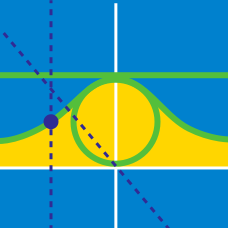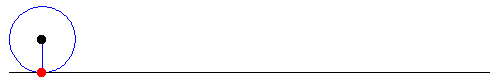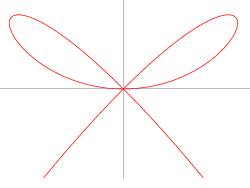Calculus

# Parametric Equations Calculus: Level 3 Challenges

If $y$ is a function of $x$ as defined by the parametric relation $y=3\sin ^{ 2 }{ t }$ when $x=\tan { t }$, then determine the value of $\displaystyle\lim_{ x\rightarrow \infty }{ y }$ .The parametric equation of a cycloid is given below.

$\large x = a(t - \sin t) \\ \large y = a(1 - \cos t)$

What is the area of the region bounded by the two arcs of the cycloid in the above figure?

What is the length of the arc of the curve $x^{\frac{2}{3}} + y^{\frac{2}{3}}=4$?The graph above satisfy the equation $x^4 + y^3 = x^2 y$.

The area enclosed by the 2 cute adorable little fine loops is equals to $\frac {a}{b}$ for coprime positive integers $a$ and $b$. What is the value of $a+b$?

The location of a dot $P$ at a given time $t$ in the $xy$ plane is given by $(x,y) = (t - \sin t, 1 - \cos t)$. What is the distance traveled by $P$ in the interval $0 \leq t \leq 2\pi$?

×